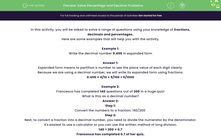# Solve Percentage and Decimal Problems

In this worksheet, students will be asked to solve worded problems using their knowledge of fractions, decimals and percentages.Key stage:  KS 2

Curriculum topic:   Number: Fractions, Decimals and Percentages

Curriculum subtopic:   Solve Percentage/Decimal Problems

Popular topics:   Place Value worksheets

Difficulty level:#### Worksheet Overview

In this activity, you will be asked to solve a range of questions using your knowledge of fractions, decimals and percentages.

Example 1:

Write the decimal number 0.456 in expanded form

Expanded form means to partition a number to see the place value of each digit clearly.

Because we are using a decimal number, we will write its expanded form using fractions.

0.456 = 4/10 + 5/100 + 6/1000

Example 2:

Francesca has completed 140 questions out of 200 in a huge quiz!

What is this as a decimal number?

Step 1:

Convert the numbers to a fraction: 140/200

Step 2:

Next, to convert a fraction into a decimal number, you need to divide the numerator by the denominator.

It's easiest to use a calculator or you can use the written method of long division.

140 ÷ 200 = 0.7

Francesca has completed 0.7 of her quiz.

Example 3:

Wayne has £85 from his savings.

He then spends 30% on a football ticket.

How much money does he have left?

Step 1: Find 1/5 of £85

85 ÷ 5 = £17

Wayne has spent £17 on a present for his Dad.

Step 2: Find 30% of £85

First find 10%

£85 ÷ 10 =£8.5

10% = £8.50

30% = £8.50 x 3

= £25.50

Step 3: Add both amounts together

£25.50 + £17 = £42.50

Step 4: Subtract the total spent from the original amount

£85 - £42.50 = £42.50

Wayne has £42.50 left.

### What is EdPlace?

We're your National Curriculum aligned online education content provider helping each child succeed in English, maths and science from year 1 to GCSE. With an EdPlace account you’ll be able to track and measure progress, helping each child achieve their best. We build confidence and attainment by personalising each child’s learning at a level that suits them.

Get started